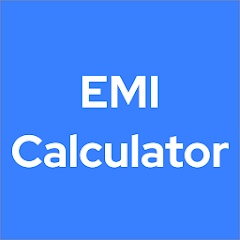# Pro Loan EMI Calculator

1K+Everyone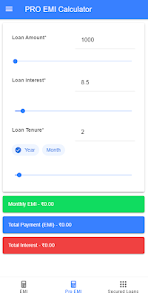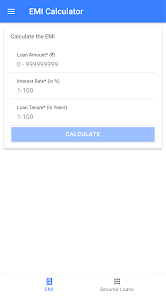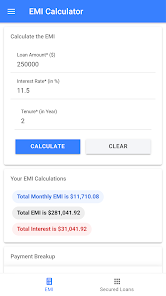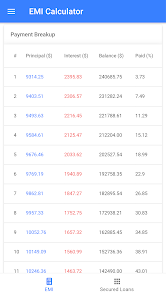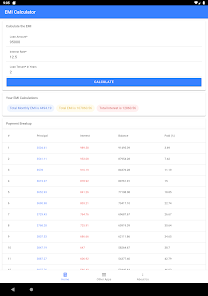Loan EMI Calculator 💰

This loan EMI calculator app simplies your EMI calculation and full break up of payment reschedule. This is an open source application hosted in public https://github.com/Aravin/emi-calculator and anyone can use the code or extend the features in the application.

The application not only displays the EMI amount, it will also display information such monthly interest paid, monthly principal paid and monthly percentage paid. It will also display payment schedule during the tenture in the tabular representation.

This is an Free / Open Soruce / Fast / Accurate / Reliable EMI calculation application.

What is EMI ?
-------------

EMI stands for Equated Monthly Iinstallment.
A fixed payment amount made by a borrower to a lender at a specified date each calendar month. Equated monthly installments are used to pay off both interest and principal each month, so that over a specified number of years, the loan is fully paid off along with interest.

How to use Loan EMI Calculation Application ❓
---------------------------------------------

Using the Loan EMI Calculation application is an very simple step. Just open the application and provider the following information in the first page i.e Total Loan amount you're going to borrow from the bank, Interest amount for the loan and tenture for the loan in terms of years and press the submit button.

Required Information:
1. Loan Amount
2. Loan Interest
3. Loan Tenure in Years

Formulas ➕
--------

In order the calculate the Loan EMI Calculation, we use following formulas
1. Interest Calculation
2. EMI Calculation
3. Percentage Calculation

Interest Calculation:

This is a formula used for Interest calculation,

interest = (principal * tenure) / (100 * 12);

EMI Calculation:

This is an formula used for EMI Calculation

E = P x r x (1+r)^n/((1+r)^n – 1)

Percentage Calculation:

This is a formula for Percentage calculation,

percentage = amount1 * 100 / amount2

## Minimum and maximum period for repayment

* Minimum Period is 3 month
* Maximum Period is 30 Years

## Maximum Annual Percentage Rate (APR)

* Min 6% and Max 45% interest

## Example EMI Calculation

Principal Amount = 150000
Rate of Interest = 10
Duration of Repayment = 3 years (36 month)

EMI = [P x R x (1+R)^N]/[{(1+R)^N}-1]

EMI = [150000 x 10/(100*12) x (1.0083)^36]/[{(1.0083)^36}-1]

= {1250 x 1.3482}/0.3482

= 4840

Accuracy & Math Error ⚡
---------------------

Our calculation and usage of formula in Loan EMI calculation will be 100% accurate and reliable. However there may be possible of 0.01% of math error because of round the number or converting the decimal number to any absolute value.

Added features to compare the loan
--------------------------------------------------------
Now, with the latest update you can compare the 2 loan accounts side by side and choose the best one of them instantly with beautify charts and detailed information.

List of Loans 🎯
-------------
1. Personal Loan EMI Calculation
4. Gold Loan EMI Calculation
5. Balance Transfer Loan EMI Calculation
6. Two Wheeler Loan EMI Calculation
7. Four Wheeler Loan EMI Calculation
9. Flexi Loan EMI Calculation
10. Student Loan EMI Calculation
13. Loan Against Car Loan EMI Calculation
14. Loan Against Equity Loan EMI Calculation
15. Loan Against Share Loan EMI Calculation
16. Loan Against Fixed Deposit Loan EMI Calculation
17. SME Loan EMI Calculation
18. MSME Loan EMI Calculation
19. Loan Against Property Loan EMI Calculation
20. Doctor Loan EMI Calculation
21. Chartered Accountants Loan EMI Calculation
22. Mortgage Loan EMI Calculation
23. Auto Loan EMI Calculation
24. Tractor Loan EMI Calculation
26. Consumer Durables Loan EMI Calculation
27. Enterprises Loan EMI Calculation
Updated on
Jul 15, 2020

## Data safety

Safety starts with understanding how developers collect and share your data. Data privacy and security practices may vary based on your use, region, and age. The developer provided this information and may update it over time.No data shared with third parties